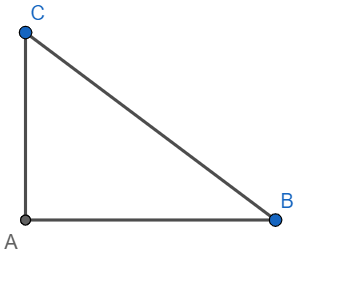QUESTION

# if $\sec \theta =2p$ and $\tan \theta =\dfrac{2}{p}$, then the value of $2\left( {{p}^{2}}-\dfrac{1}{{{p}^{2}}} \right)$ is[a] $\dfrac{1}{2}$[b] $\dfrac{1}{\sqrt{2}}$[c] $\dfrac{1}{4}$[d] $\dfrac{1}{8}$

Hint: Form a triangle which satisfies $\tan \theta =\dfrac{2}{p}$. Now using this find the value for $\sec \theta$. Hence form an equation in p and hence find the value of $2\left( {{p}^{2}}-\dfrac{1}{{{p}^{2}}} \right)$. Alternatively, use ${{\sec }^{2}}x-{{\tan }^{2}}x=1$.

Trigonometric ratios:
There are six trigonometric ratios defined on an angle of a right-angled triangle, viz sine, cosine,
tangent, cotangent, secant and cosecant.
The sine of an angle is defined as the ratio of the opposite side to the hypotenuse.
The cosine of an angle is defined as the ratio of the adjacent side to the hypotenuse.
The tangent of an angle is defined as the ratio of the opposite side to the adjacent side.
The cotangent of an angle is defined as the ratio of the adjacent side to the opposite side.
The secant of an angle is defined as the ratio of the hypotenuse to the adjacent side.
The cosecant of an angle is defined as the ratio of the hypotenuse to the adjacent side.
Observe that sine and cosecant are multiplicative inverses of each other, cosine and secant are
multiplicative inverses of each other, and tangent and cotangent are multiplicative inverses of each
other.
We have ${{\sin }^{2}}x+{{\cos }^{2}}x=1,{{\sec }^{2}}x=1+{{\tan }^{2}}x$ and ${{\csc }^{2}}x=1+{{\cot }^{2}}x$. These identities are called Pythagorean identities.Let AC = 2 and AB = p
Using Pythagoras theorem, we have
\begin{align} & \text{B}{{\text{C}}^{2}}=\text{A}{{\text{B}}^{2}}\text{+A}{{\text{C}}^{2}} \\ & \Rightarrow \text{B}{{\text{C}}^{2}}={{2}^{2}}+{{p}^{2}}=4+{{p}^{2}} \\ \end{align}
Now we have
\begin{align} & \sec \theta =2p \\ & \Rightarrow {{\sec }^{2}}\theta =4{{p}^{2}} \\ \end{align}
We know that the secant of an angle is the ratio of the hypotenuse to the adjacent side.
Hence ${{\sec }^{2}}\theta ={{\left( \dfrac{\text{BC}}{\text{AB}} \right)}^{2}}=\dfrac{4+{{p}^{2}}}{{{p}^{2}}}=\dfrac{4}{{{p}^{2}}}+1$
Hence, we have
\begin{align} & \dfrac{4}{{{p}^{2}}}+1=4{{p}^{2}} \\ & \Rightarrow 4{{p}^{2}}-\dfrac{4}{{{p}^{2}}}=1 \\ & \Rightarrow 4\left( {{p}^{2}}-\dfrac{1}{{{p}^{2}}} \right)=1 \\ \end{align}
Hence, we have $2\left( {{p}^{2}}-\dfrac{1}{{{p}^{2}}} \right)=\dfrac{1}{2}$
Hence option [a] is correct.

Note: Alternative Method: Best Method.
We have $\sec \theta +\tan \theta =2p+\dfrac{2}{p}$
and $\sec \theta -\tan \theta =2p-\dfrac{2}{p}$
Multiplying, we get
\begin{align} & \left( \sec \theta -\tan \theta \right)\left( \sec \theta +\tan \theta \right)=\left( 2p+\dfrac{2}{p} \right)\left( 2p-\dfrac{2}{p} \right) \\ & \Rightarrow {{\sec }^{2}}\theta -{{\tan }^{2}}\theta =4{{p}^{2}}-\dfrac{4}{{{p}^{2}}} \\ & \Rightarrow 4{{p}^{2}}-\dfrac{4}{{{p}^{2}}}=1 \\ & \Rightarrow 2\left( {{p}^{2}}-\dfrac{1}{{{p}^{2}}} \right)=\dfrac{1}{2} \\ \end{align}
Hence option [a] is correct.